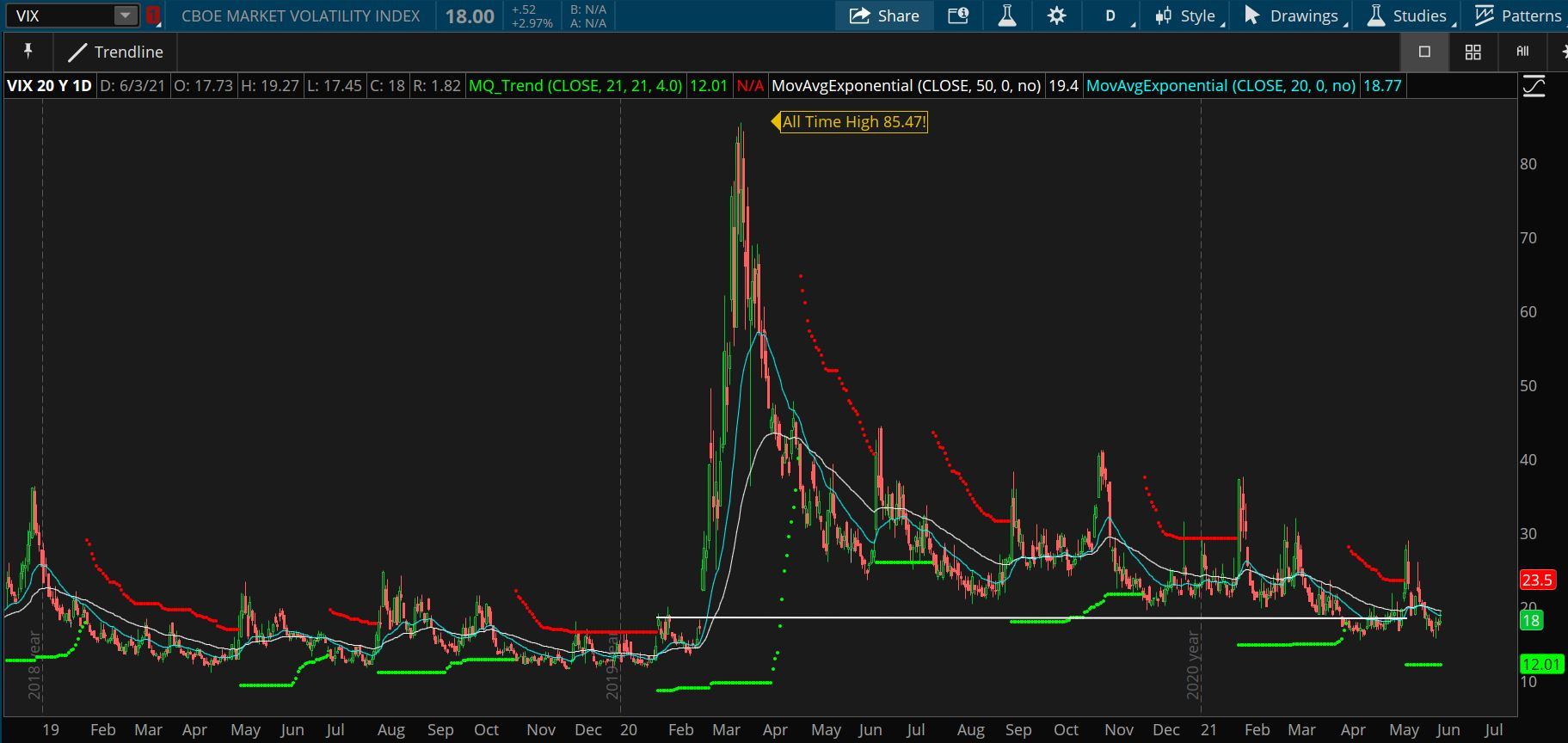Volatility is the most common way to measure risk in the financial markets. While there are a plethora of methods, calculations and derivatives to calculate volatility, they are all trying to accomplish the same goal: What is the price of a security going to do in the future? Without a crystal ball, there’s no perfect answer, but let’s go through a few common ways that we can estimate future volatility.

Generally speaking, there are two types of volatility traders and investors use in an effort to understand risk – historical volatility and implied volatility. Each of these can be used in different ways for different types of trades. Today, we’re going to go through the basics of implied volatility, starting with the .

First proposed as the Sigma Index in 1987, the VIX got its start in 1993 when the CBOE reported implied volatility in real time using at-the-money options data from the S&P 100. While updates have been made to the VIX over time, it’s used as a “fear gauge,” or a measure of the market’s expectations of future price action. As the VIX increases, the market expects more risk ahead.Take a look at the most recent recession in 2020 – where we saw the VIX climb as high as 85. At that price, the market is expecting extreme risk. Ultimately, the VIX is the industry standard to help traders and investors have a standardized view of market risk through implied volatility.

The VIX is useful because it can give us a hint at what the market is expecting since it is an example of implied volatility. Typically, IV is derived using an options pricing model, such as the Black–Scholes. Using these models, the theoretical value of an option can help guide us to the measurement of implied volatility at a particular point in time.

Traders and investors can use IV to find attractive options trades to hedge, enter or exit a position, or to speculate on a future outcome in the market.

## Historical Volatility

While the VIX is a measure of implied volatility, there are many historical measures of volatility that can be useful. One common example is the beta coefficient. This is a historical calculation measured by taking the returns associated with a security and comparing the price action of the market over the same time period. A security with a beta less than 1 implies that the security is theoretically less volatile than the market as a whole. A security with a beta greater than 1 would be more volatile than the market.

For those that want to have a full picture of the risk of a security, the beta coefficient can help separate market risk from individual security risk. These types of measures can help you diversify properly with respect to your individual risk tolerance.

A historical volatility calculation like beta gives you a basic understanding of what the price of a security has done in the past. While past performance is not indicative of future results, historical volatility calculations can be used to help measure risk and ultimately help determine if a security is right for you.

## The Volatility Of The Volatility – VVIX

To further confuse new traders there is such a thing called the volatility of the volatility – AKA the VIX of the VIX (VVIX). No this is not an exercise in doublespeak. The VVIX is simply a measure of the change of volatility in the VIX volatility index. The VVIX is the VIX of the VIX, like the VIX is the VIX of stocks. OK, if you are not thoroughly confused by now then congrats because this stuff can get pretty mind-bending! Another way to put it is, the VVIX measures how rapidly volatility changes, and is, thus, a measure of the volatility of the index. Investors can use the VVIX and its derivatives to hedge against volatility swings on changes in the VIX options market. You can hedge the hedge!

## Why You Shouldn’t Be Afraid Of Volatility

All told, volatility is just a measurement that can give you insight into the potential risk of a security. It’s important to remember that actual volatility is almost always less than implied volatility. No measure of risk is going to be totally accurate, anything can happen in the financial markets. Even so, volatility measurements can offer a clear view of risk the market expects.